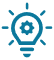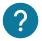# What is a list? How to create and use one?

## Introduction

A list allows you to contain several values in order to store them and reuse them in your program. Lists are declared differently depending on whether they are integers, strings or floats.### Variable or list?

If you want to store a single value, you can use a variable instead of a list. Here are our lessons on variables, integers, strings or float.

## Creating a list

We will now see how to create a list of values. For this you have two possibilities: You can create a list with the values directly or just give the size of the list and assign the values later in the program.

a) List created with values

Here is how to create a list with the values already in it:

``int my_list_integer={9, 3, 2, 4, 3, 2, 7, 8, 9, 11};``

These values can be changed later in your program. You can look at the list operation section to see how to do this.

b) List created without values

``int my_list_integer;``

Here we have just created a list with 6 places available to store 6 integers. You don’t have to assign 6 values at once, but you can’t put 7 for example.

Be careful, if you assign values, be aware that the last value does not count because the 0 is also counted. For example, if you want to assign a value for a list of 6 values, you can take 0,1,2,3,4,5.

Here is how you add a value to this list:

``````int my_list_integer; // We create the list
void setup() {
my_list_integer == 2 // We add 2 in the list
}

void loop() {
}``````

We will see in the operations on the lists section how to retrieve a value from a list.

The example we have just given works for lists of integers. However, it also works for lists of decimal numbers and strings. Nevertheless there are small differences:

b) List of decimal numbers

Here is how to create a list of decimal numbers:

``float my_list_decimal={9.2, 3.1, 2.0, 4.7, 3.2, 2.9, 7.4, 8.1, 9.0, 11.4};``

c) Character string list

Here is how to create a string list with the values already in it:

``char my_list_string = "arduino_factory";``### Can we put integers and strings in the same list?

A list must contain only the type of data you have specified. For example, if you have created an integer list, you cannot add decimal numbers or strings.

## Operation on the lists

We will now see how to do operations on lists, and assign values and retrieve a value in a variable.

a) Assign a value in the list

In this part we will see how to assign a value in a list or change a value in a list.

Here is a list of integers where we change the second value:

``````int my_list_integer; // We create a list

void setup() {
my_list_integer == 2; // We assign the value 2 in the list
}

void loop() {
}``````

To change the value of a list, it’s exactly the same lines of code as for assigning a value. You just have to change the number of the list where you want to assign a value.

b) How to retrieve a value in a variable

We will now see how to retrieve one of the values from the list:

``int my_variable = my_list_integer;// To retrieve the second number of the list if that one exist``

c) Know the size of your list

We will now see how to know the size of your list:

``int variable_size = sizeof(your_list_integer);``

The size of the list is contained in variable_size.

d) Display all the values in the list

We will now see how to display all the values of a list:

``````for (byte i = 0; i < 6; i = i + 1) { // The loop goes from 0 to 6
Serial.println(votre_liste_entier[i]); // We display each elements of the list
}``````